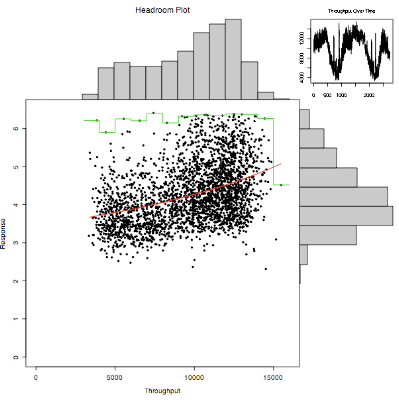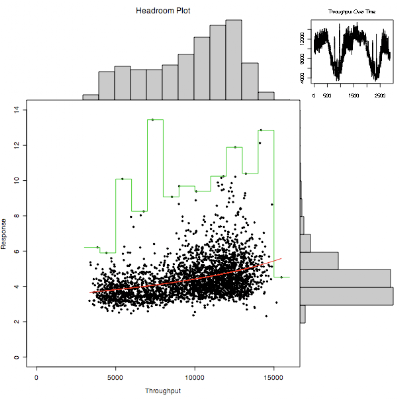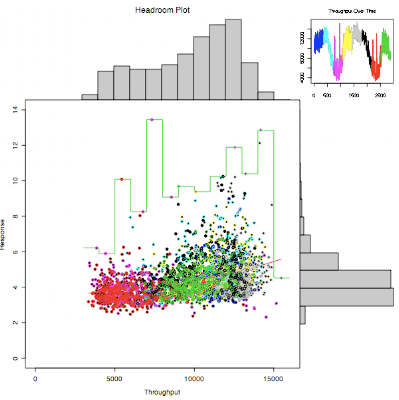## Monday, July 28, 2008

About eighteen months ago I wrote a blog post about Skype as it approached 500 million downloads. They have continued at around a million a day, and now, nearly 500 days later we are coming up on the one billion mark. I predict at the current rate that the last 42 million downloads will happen in 37 days, so it will pass a billion around September 3rd.

The usual caveats apply, most of the downloads are version upgrades, but there are over 300 million different registered skype names, and concurrent user counts peak at 12 million most days.

At the time I got a nice mention from Skype Journal, and you can find good analysis of the Skype usage levels at Jean Mercier's Skype Numerology blog. As ever, you can track the actual numbers via an RSS feed that Skype provides, or just view them at this graph.

Why does this matter? It matters because this is the kind of critical mass that the rest of the Telco industry and VOIP startups are only dreaming of. I'm about to turn off my long distance and international phone plans and just have local calls on my home phone. The home phone I just ordered will redirect long distance and international calls over Skype, and make local calls on the land line. This will save me hundreds of dollars a year in telco service costs, so it pays for itself in less than six months. There is even a special offer on the hardware right now. I fully expect that in a few years time I will also be making the bulk of my mobile calls over Skype as well.

All I want from the Telco's is reliable unlimited raw bandwidth at a fixed low price, which is a terrifying prospect for the dinosaurs of this industry...

## Monday, July 14, 2008

### Enhanced headroom plot in R

For some reason I seem to find time to write code in R when I'm on an airplane. The last two trips I made resulted in significant enhancements and debugging of the code for my headroom plot. It started off simple but it now has a lot of bells and whistles, including color coding.

Main changes: the quantile used to remove outliers now only removes outliers that exceed the 95th percentile response time by default. It keeps all the throughput values unless you use qx=True.

In each of the throughput bins used to draw the histogram, the maximum response time for that bin is now calculated and displayed as a staircase line unless you set max=False.

The set of data is now split into ranges and color coded. The times series plot is coded so you can see the split, and the scatterplot shows how those points fall. I have been plotting weekly data at one minute intervals with split=7, which looks pretty good.

I read in some data that has been extracted from vxstat into a csv format at a known URL and plotted it three ways.
I plot the first 2880 data points, picking the read data rather than write, two days at one minute intervals.

`> stime <- read.csv(url("http://somewhere/vxstat_response"))> sops <- read.csv(url("http://somewhere/vxstat_throughput"))> names(sops) "DateTime"                             "vxstat_dg_operationsRead"  "vxstat_dg_operationsWrite"> chp(sops[1:2880,2],stime[1:2880,2])> chp(sops[1:2880,2],stime[1:2880,2],q=1.0)> chp(sops[1:2880,2],stime[1:2880,2],q=1.0,splits=8)`Here is the code that generates the plot.

`> chp <-function(throughput,response, q=0.95, qx=F, xl="Throughput",yl="Response",tl="Throughput Over Time",ml="Headroom Plot", fit=T, max=T, splits=0) {      # remove zero throughput and response values      nonzer <- (throughput != 0) & (response != 0)  # array of true/false      y <- response[nonzer]      x <- throughput[nonzer]      # remove outliers, keep response time points inside 95% by default      if (q != 1.0) {              quant <- (y < quantile(y,q))              # optionally trim throughput outliers as well              if (qx) quant <- quant & (x < quantile(x, q))              x <- x[quant]              y <- y[quant]      }      # make histograms and record end points for scaling      xhist <- hist(x,plot=FALSE)      yhist <- hist(y,plot=FALSE)      xbf <- xhist\$breaks                          # first      ybf <- yhist\$breaks                          # first      xbl <- xhist\$breaks[length(xhist\$breaks)]       # last      ybl <- yhist\$breaks[length(yhist\$breaks)]       # last      xcl <- length(xhist\$counts)                     # count length      ycl <- length(yhist\$counts)                     # count length      xrange <- c(0.0,xbl)      yrange <- c(0.0,ybl)      xlen <- length(x)      # make a multi-region layout      nf <- layout(matrix(c(1,3,4,2),2,2,byrow=TRUE), c(3,1), c(1,3), TRUE)      layout.show(nf)      # set plot margins for throughput histogram and plot it      par(mar=c(0,4,3,0))      barplot(xhist\$counts, axes=FALSE,              xlim=c(xcl*0.00-xbf/((xbl-xbf)/(xcl-0.5)),xcl*1.00),              ylim=c(0, max(xhist\$counts)), space=0, main=ml)      # set plot margins for response histogram and plot it sideways      par(mar=c(5,0,0,1))      barplot(yhist\$counts, axes=FALSE, xlim=c(0,max(yhist\$counts)),              ylim=c(ycl*0.00-ybf/((ybl-ybf)/(ycl-0.5)),ycl*1.00),              space=0, horiz=TRUE)      # set plot margins for time series plot      par(mar=c(2.5,1.7,3,1))      plot(x, main=tl, cex.axis=0.8, cex.main=0.8, type="S")      if (splits > 0) {   step <- xlen/splits   for(n in 0:(splits-1)) {    lines((1+n*step):min((n+1)*step,xlen), x[(1+n*step):min((n+1)*step,xlen)], col=4+n)   }      }      # set plot margins for main plot area      par(mar=c(5,4,0,0))      plot(x, y, xlim=xrange, ylim=yrange, xlab=xl, ylab=yl, pch=20)      if (max) {        # max curve        b <- xhist\$breaks        i <- b - b # interval        maxl <- list(y[b < x & x <= (b+i)])        for(n in b[c(-1,-length(b))]) maxl <- c(maxl,list(y[n < x & x <= (n+i)]))        #print(maxl)        maxv <- unlist(lapply(maxl,max)) # apply max function to elements of list        #print(maxv)        #lines(xhist\$mids,maxv,col=2)  # join the dots        #staircase plot showing the range for each max response        lines(rep(b,1,each=2)[2:(2*length(maxv)+1)],rep(maxv,1,each=2),col=3)              }      if (fit) {        # fit curve, weighted to predict high throughput        # create persistent chpfit object using <<-        chpfit <- glm(y ~ x, inverse.gaussian, weights=as.numeric(x))        # add fitted values to plot, sorted by throughput        lines(x[order(x)],chpfit\$fitted.values[order(x)],col=2)      }      if (splits > 0) {   step <- xlen/splits   for(n in 0:(splits-1)) {    Sys.sleep(1)    points(x[(1+n*step):min((n+1)*step,xlen)],y[(1+n*step):min((n+1)*step,xlen)], xlim=xrange, ylim=yrange, col=4+n)   }      }}`

### iPhone 2.0 upgrade and applications - Pandora

I waited until Saturday evening to attempt an upgrade on my iPhone, and it completed with no problems, so I guess Apple fixed their site and the rush had died down a bit.

I looked through some of the applications and loaded Pandora, eBay, Paypal (for old times sake), Weatherbug, Remote (I also upgraded my AppleTV so this will come in useful) and Facebook. Enough to get me started. I also saw a nice star mapping application, I used to have one of these on my Palm/Treo but it wasn't free, so I decided to leave it for later.

The Pandora application is my favorite. I am an intermittent user of Pandora, as I didn't like being tethered to my laptop to listen. However the iPhone version works fine even over a fairly poor EDGE signal. There are some pauses between songs and the occasional pause during a song, but overall its a nice way to listen to the channels I had already setup, and discover new music that is similar to my favorites. I suspect that this is going to get a lot of usage since I can listen to it on the go.

There are over 500 applications, so plenty of stuff to play with if I get bored.

I applied for iPhone developer program membership when it was announced, and got an email yesterday saying that I have been accepted, so went through the purchase process, and downloaded the final version of the iPhone SDK....